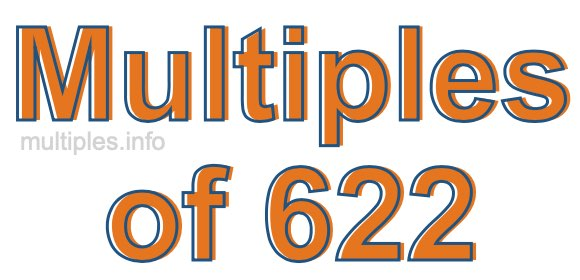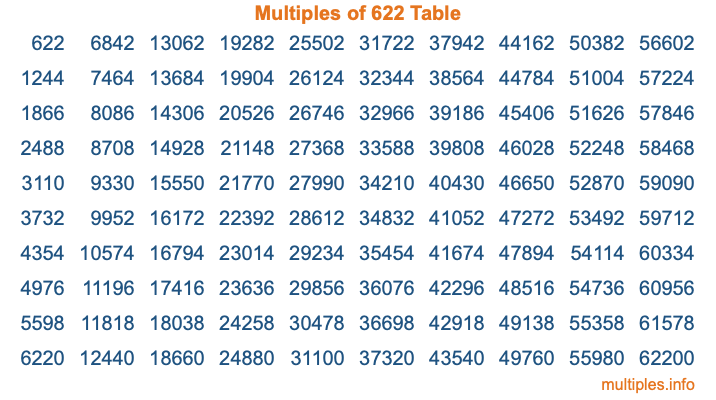Multiples of 622Welcome to the Multiples of 622 page. Here we will first teach you everything you will ever need to know about the multiples of 622, and then give you a study guide summary of everything we taught you to make sure you remember it all. Use this page to look up facts and learn information about the multiples of 622. This page will make you a multiples of six hundred twenty-two expert!

Definition of Multiples of 622
Multiples of 622 are all the numbers that when divided by 622 equal an integer. Each of the multiples of 622 are called a multiple. A multiple of 622 is created by multiplying 622 by an integer.

Therefore, to create a list of multiples of 622, you start with 1 multiplied by 622, then 2 multiplied by 622, then 3 multiplied by 622, and so on for as long as you want. Thus, the list of the first five multiples of 622 is 622, 1244, 1866, 2488, and 3110. To see a larger list of multiples of 622, see the printable image of Multiples of 622 further down on this page. We also have a category where you can choose any nth multiple of 622.

Multiples of 622 Checker
The Multiples of 622 Checker below checks to see if any number of your choice is a multiple of 622. In other words, it checks to see if there is any number (integer) that when multiplied by 622 will equal your number. To do that, we divide your number by 622. If the the quotient is an integer, then your number is a multiple of 622.

Is  a multiple of 622?

Least Common Multiple of 622 and ...
A Least Common Multiple (LCM) is the lowest multiple that two or more numbers have in common. This is also called the smallest common multiple or lowest common multiple and is useful to know when you are adding our subtracting fractions. Enter one or more numbers below (622 is already entered) to find the LCM.

Check out our LCM Calculator if you need more details about the Least Common Multiple or if you need the LCM for different numbers for adding and subtraction fractions.

nth Multiple of 622
As we stated above, 622 is the first multiple of 622, 1244 is the second multiple of 622, 1866 is the third multiple of 622, and so on. Enter a number below to find the nth multiple of 622.

th multiple of 622

Multiples of 622 vs Factors of 622
622 is a multiple of 622 and a factor of 622, but that is where the similarities end. All postive multiples of 622 are 622 or greater than 622. All positive factors of 622 are 622 or less than 622.

Below is the beginning list of multiples of 622 and the factors of 622 so you can compare:

Multiples of 622: 622, 1244, 1866, 2488, 3110, etc.

Factors of 622: 1, 2, 311, 622

As you can see, the multiples of 622 are all the numbers that you can divide by 622 to get a whole number. The factors of 622, on the other hand, are all the whole numbers that you can multiply by another whole number to get 622.

It's also interesting to note that if a number (x) is a factor of 622, then 622 will also be a multiple of that number (x).

Multiples of 622 vs Divisors of 622
The divisors of 622 are all the integers that 622 can be divided by evenly. Below is a list of the divisors of 622.

Divisors of 622: 1, 2, 311, 622

The interesting thing to note here is that if you take any multiple of 622 and divide it by a divisor of 622, you will see that the quotient is an integer.

Multiples of 622 Table
Below is an image of the first 100 multiples of 622 in a table. The table is in chronological order, column by column. The first column has the first ten multiples of 622, the second column has the next ten multiples of 622, and so on.The Multiples of 622 Table is also referred to as the 622 Times Table or Times Table of 622. You are welcome to print out our table for your studies.

Negative Multiples of 622
Although not often discussed or needed in math, it is worth mentioning that you can make a list of negative multiples of 622 by multiplying 622 by -1, then by -2, then by -3, and so on, to get the following list of negative multiples of 622:

-622, -1244, -1866, -2488, -3110, etc.

Multiples of 622 Summary
Below is a summary of important Multiples of 622 facts that we have discussed on this page. To retain the knowledge on this page, we recommend that you read through the summary and explain to yourself or a study partner why they hold true.

There are an infinite number of multiples of 622.

A multiple of 622 divided by 622 will equal a whole number.

622 divided by a factor of 622 equals a divisor of 622.

The nth multiple of 622 is n times 622.

The largest factor of 622 is equal to the first positive multiple of 622.

622 is a multiple of every factor of 622.

622 is a multiple of 622.

A multiple of 622 divided by a divisor of 622 equals an integer.

622 divided by a divisor of 622 equals a factor of 622.

Any integer times 622 will equal a multiple of 622.

Multiples of a Number
Here you can get the multiples of another number, all with the same attention to detail as we did for multiples of 622 on this page.

Multiples of
Multiples of 623
Did you find our page about multiples of six hundred twenty-two educational? Do you want more knowledge? Check out the multiples of the next number on our list!